## Monday, 20 February 2012

### IB Maths Studies

The blog post is just to give an idea of some of the things IB Maths Studies students do here at IST. The theme here is 'Enquiry based learning' and by that I am talking about the sorts of activities that provide opportunities for students to make discoveries on their own through engagement induced enquiry! At least that is the aim! Let explain the point with the following examples The title each links to a fuller, resourced, outline of the activity.

Probability treesThis activity is about bridging the gap between the intuition of sample space diagrams and the efficiency of tree diagrams. Students will look at a problem from the two points of view, play with multiplying and adding fractions and hopefully see how tree diagrams are a more efficient way of doing the same thing! The activity is good for group work and physical manipulation, although it could be completed on computers by individuals if required. It may well take 2 to 3 hours to complete all of the tasks, but at the end, the hope is that students have a strong understanding of how tree diagrams work that they can apply to different problems.

ScattertasticThis activity makes use of two excellent virtual manipulative that are freely available on the web. The activity helps students to begin understanding the concepts of correlation, lines of best fit and degrees of correlation through the use of these manipulative.

Meeting Functions - Challenge students to really understand the concept of a function. Match a set of input values with a function and a corresponding set of output values. There are eight sets of three to make and only one correct solution. This activity is 'old meets new'. Students work with cut out bits of paper but can use calculators/computers to help them solve the puzzle!

Quadratic Links - This activity is about linking the graphing of quadratics with the equations themselves by looking at their key features. Students match pieces of information with different graphs using logical deduction. This practical group activity leads to being able to sketch graphs from their equations.

Making Cones - Explore cones by making one! This activity helps students understand where the formula for the surface area of a cone comes from and play with the associated mathematics. A great practical task that seems easy and works out to be more of a challenge. In making the cone students will confront some great mathematical reasoning and maybe even some algebraic proof!

Which Rule - This activity is designed to help students solve trigonometry problems by encouraging them to 'Speculate' about what might be possible. Students are asked to state different truths or complete different equations for a given diagram without being told what to solve for. Having completed the equations they are asked to think about which of them is most useful for solving for a particular variable. So often students feel that they must know 'the right thing to do' before they proceed and are afraid to try things out to see what happens. Yes, it is possible to learn how to recognise certain types of problems but it is equally important to learn that problems can be solved by trying to use the different pieces of knowledge you have to make new ones. The sine rule and cosine rule can both be applied to any given triangle it is just that often only one of them generates an equation that can be solved. We can either learn to spot types of problems or to speculate with both. In practice, one often leads to the other and then we are better equipped to solve more problems.

1.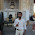ib mathematics We porvide IB Math Portfolio & IB Math Portfolio Sample on student's request. You can get portfolio instantly after your request ib chemistry

2.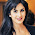ib math sl Chemistry is a very important subject and is essential if you are planning to read medicine, dentistry or pharmacy at university. At A-Level this subject is highly regarded by universities and employers. If you have not decided upon a career path chemistry may be a good option as it leaves many doors open. If you require extra help with this subject please contact one of our chemistry tutors below. ib math studies

3.ib math We porvide IB Math Portfolio & IB Math Portfolio Sample on student's request. You can get portfolio instantly after your request. ib math studies

4.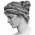Very interesting article!!
I'd like to comment that MYP and IB (HL,SL,Math Studies) students maybe find helpful the IB math forum at IB maths 4u
Thanks!

5.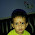Get highly experienced IB & IGCSE Maths tutors, IB Maths, IB Maths Tutor in Gurgaon, Delhi, Noida. Our tutors are experts in IB, A/AS Level, AP & SAT, and IGCSE Maths Tuition.

6.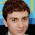IB math is a main subject and essential also, So IB global academy provides facility to those students whose are weeker and not good. We provides the classes of Maths Tutor, IB Maths Tutor , IGCSE Maths Tuition, IB Maths Tutor in Gurgaon, Maths Tutor Delhi, IB Maths, IGCSE Maths Tutor in Gurgaon, IB Home Tutor in Gurgaon, IB Home Tutor in Delhi, Home Tuition in Gurgaon and IB Tutor In Delhi etc.